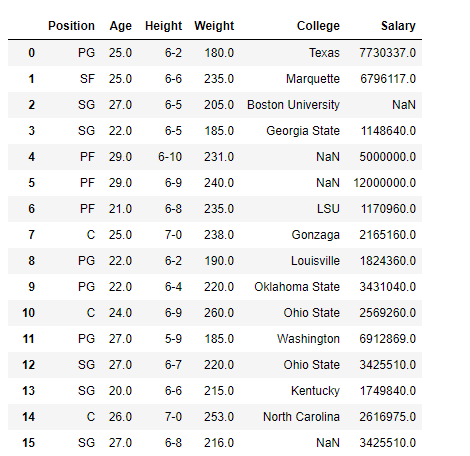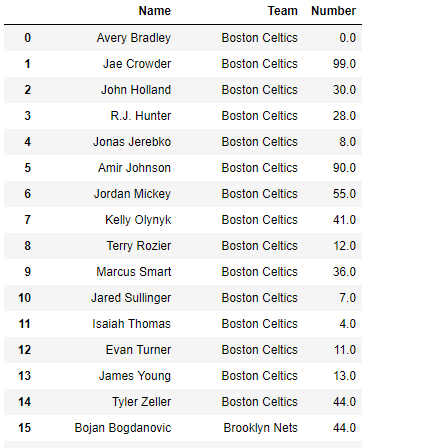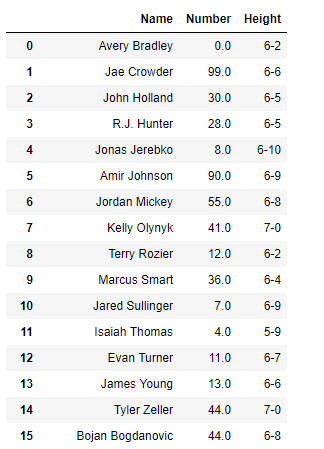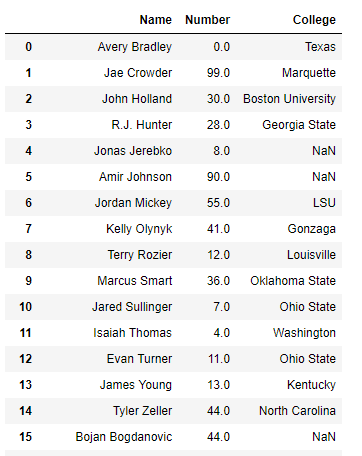Open in App
Not now

# Creating views on Pandas DataFrame

• Last Updated : 23 Jan, 2019

Many times while doing data analysis we are dealing with a large data set, having a lot of attributes. All the attributes are not necessarily equally important. As a result, we want to work with only a set of columns in the dataframe. For that purpose, let’s see how we can create views on the Dataframe and select only those columns that we need and leave the rest.

Solution #1: A set of columns in the DataFrame can be selected by dropping all those columns which are not needed.

 `# importing pandas as pd``import` `pandas as pd`` ` `# Reading the csv file``df ``=` `pd.read_csv(``"nba.csv"``)`` ` `# Print the dataframe``print``(df)`

Output :Now we will select all columns except the first three columns.

 `# drop the first three columns``df.drop(df.columns[[``0``, ``1``, ``2``]], axis ``=` `1``)`

Output :We can also use the names of the column to be dropped.

 `# drop the 'Name', 'Team' and 'Number' columns``df.drop([``'Name'``, ``'Team'``, ``'Number'``], axis ``=` `1``)`

Output :Solution #2 : We can individually select all those columns which we need and leave out the rest.

 `# importing pandas as pd``import` `pandas as pd`` ` `# Reading the csv file``df ``=` `pd.read_csv(``"nba.csv"``)`` ` `# select the first three columns``# and store the result in a new dataframe``df_copy ``=` `df.iloc[:, ``0``:``3``]`` ` `# Print the new DataFrame``df_copy`

Output :We can also select the columns in a random manner by passing a list to the `DataFrame.iloc` attribute.

 `# select the first, third and sixth columns``# and store the result in a new dataframe``# The numbering of columns begins from 0``df_copy ``=` `df.iloc[:, [``0``, ``2``, ``5``]]`` ` `# Print the new DataFrame``df_copy`

Output :Alternatively, we can also name the columns that we want to select.

 `# Select the below listed columns``df_copy ``=` `df[[``'Name'``, ``'Number'``, ``'College'``]]`` ` `# Print the new DataFrame``df_copy`

Output :My Personal Notes arrow_drop_up